Vectors and conics

This free course is available to start right now. Review the full course description and key learning outcomes and create an account and enrol if you want a free statement of participation.

Free course

2.7 Further exercises

Example 31

Let p = 2i − 3j + k and q = −i −2j −4k be two vectors in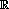3. Determine p + q, pq and 2p − 3q.

Since p = 2i − 3j + k and q = −i −2j −4k, we have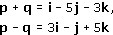and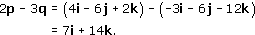Example 32

Let u and v be the position vectors (1, 1) and (2, 1), respectively.

• (a) Determine the position vectors u + 2v, −u, −u + 3v and u − 3v.

• (b) On a single diagram, sketch u, v and the position vectors that you found in part (a).

• (a) Here,

•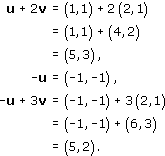• and

•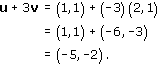• (b)

•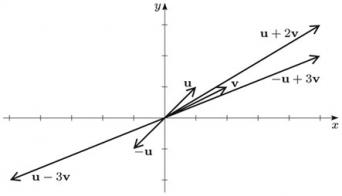Example 33

Let u = (2, 6) and v = (4, 2).

• (a) Determine numbers α and β such that

•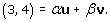• Hint: Obtain simultaneous equations involving α and β by equating the first and second components of the vectors on each side of equation (2.9).

• (b) Sketch the position vectors u, v and (3, 4) on a single diagram.

• (a)First we write

•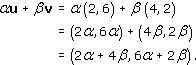• Thus

•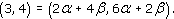• Then, following the hint, we equate the first and second components of these vectors; this gives two simultaneous equations:

•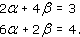• These two equations can be solved to give

•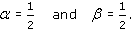• Thus we can write

•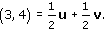• (b)

•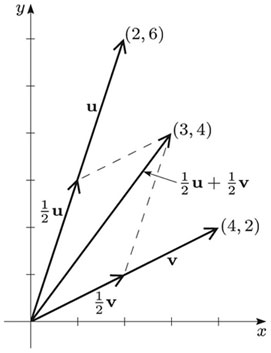Example 34

Let A and B be the points (5, 4) and (−2, −3).

Determine the point R that divides AB in the ratio 2 : 5, and the midpoint M of AB.

The point R divides AB in the ratio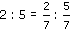. It follows from the Section Formula, with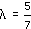, that the position vector r of R is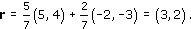Hence the coordinates of R are (3, 2).

The midpoint M of AB has position vector m, where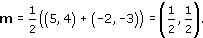Hence the coordinates of M are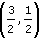.

Example 35

• (a) Determine the vector form of the equation of the line ℓ through the points (2, 3) and (5, −1).

• (b) Hence determine whether the points (7, 2) and (−1, 7) lie on ℓ.

• (a) Let p = (2, 3) and q = (5, −1). Then the vector form of the equation of the line ℓ is

•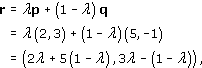• that is,

•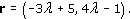• where λ ∈.

• (b) The point (7, 2) lies on the line ℓ if there is a value of λ such that

•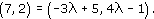• Equating components in turn, we obtain

•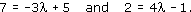• The second equation gives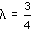, but this value of λ does not satisfy the first equation. It follows that (7, 2) does not lie on the line ℓ.

• The point (−1, 7) lies on the line ℓ if there is a value of λ such that

•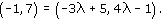• Equating components in turn, we obtain

•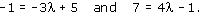• The second equation gives λ = 2, and this value also satisfies the first equation. It follows that the point (−1, 7) lies on the line ℓ.

Example 36

The rectangle OABC has vertex O at the origin, and the position vectors of the vertices A, B and C are a, b and c, respectively. P is the midpoint of BC, and the point Q is one-third of the way along AB from A. S is the point of intersection of the lines OC and QP.

• (a) Determine the position vectors of P and Q, in terms of a and c.

• (b) Determine the vector form of the equation of the line that passes through P and Q, in terms of a and c.

• (c) Use the equation that you obtained in part (b), and the fact that S lies on the line OC, to find the position vector of S, in terms of a and c.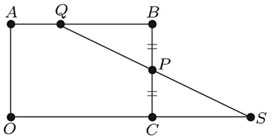• (a)P is the midpoint of BC, so its position vector p can be written as

•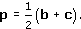• Now b = a + c by the Parallelogram Law, since OB is the diagonal of the parallelogram with adjacent sides a and c. Thus

•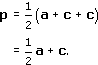• Next, the position vector q of the point Q is given by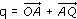. Here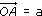; also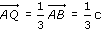, since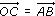(as they have the same magnitude and direction). Hence

•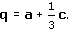• (b) The vector form of the line through P and Q is

•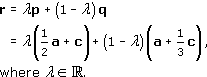• Multiplying out and collecting terms, we obtain

•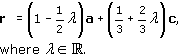• where λ ∈.

• (c) The point S lies on QP, so its position vector s can be written in the form

•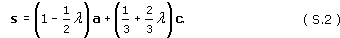• But s can also be written solely as a multiple of c, since S is also on OC and so s is in the same direction as c. This means that the coefficient of a in equation (S.2) for s must be 0.

• Thus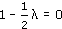, so λ = 2.

• It follows that

•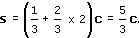M208_1